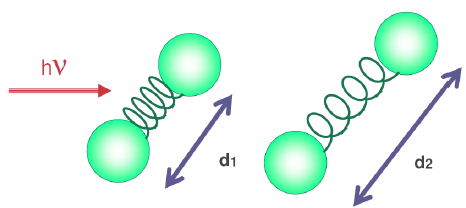The harmonic oscillator is a physical system in which a mass oscillates around an equilibrium position subjected to an elastic potential $V(x)=\frac{1}{2}kx^2$. The oscillation of the atoms in a molecule can be studied using the quantum version of this model. Solving the Schrödinger equation gives us the energy levels and the wave function.